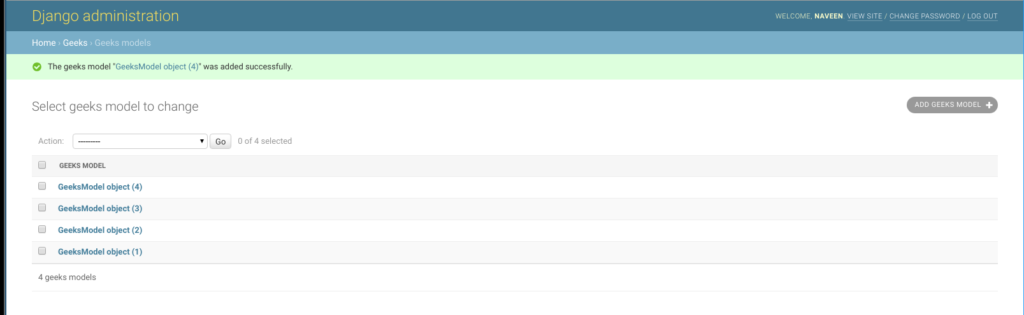# BigAutoField – Django Models

• Last Updated : 24 Aug, 2021

BigAutoField is a 64-bit integer, much like an AutoField except that it is guaranteed to fit numbers from 1 to 9223372036854775807. One can create a BigAutoField using the following syntax,

`id = models.BigAutoField(primary_key=True, **options)`

This is an auto-incrementing primary key just like AutoField.

## Django Model BigAutoField Explanation

Illustration of BigAutoField using an Example. Consider a project named geeksforgeeks having an app named geeks.

Refer to the following articles to check how to create a project and an app in Django.

Enter the following code into models.py file of geeks app.

## Python3

 `from` `django.db ``import` `models``from` `django.db.models ``import` `Model``# Create your models here.` `class` `GeeksModel(Model):``    ``big_id ``=` `models.BigAutoField(primary_key ``=` `True``)`

Add the geeks app to INSTALLED_APPS

## Python3

 `# Application definition` `INSTALLED_APPS ``=` `[``    ``'django.contrib.admin'``,``    ``'django.contrib.auth'``,``    ``'django.contrib.contenttypes'``,``    ``'django.contrib.sessions'``,``    ``'django.contrib.messages'``,``    ``'django.contrib.staticfiles'``,``    ``'geeks'``,``]`

Now when we run makemigrations command from the terminal,

`Python manage.py makemigrations`

A new folder named migrations would be created in geeks directory with a file named 0001_initial.py

## Python3

 `# Generated by Django 2.2.5 on 2019-09-25 06:00` `from` `django.db ``import` `migrations, models` `class` `Migration(migrations.Migration):` `    ``initial ``=` `True` `    ``dependencies ``=` `[``    ``]` `    ``operations ``=` `[``        ``migrations.CreateModel(``            ``name ``=``'GeeksModel'``,``            ``fields ``=``[``                ``(``'big_id'``,``                  ``models.BigAutoField(auto_created ``=` `True``,``                  ``primary_key ``=` `True``,``                  ``serialize ``=` `False``,``                  ``verbose_name ``=``'ID'``                ``)),``            ``],``        ``),``    ``]`

Thus, a big_id BigAutoField that auto increments on every instance of that model is created when you run makemigrations on the project. It is a primary key to the table created for the model named GeeksModel.

Possible Error
since a Django model can have at most one autofield, BigAutoField must be created at the very first migration with primary_key=True attribute otherwise it will raise multiple errors.

“AssertionError: A model can’t have more than one AutoField.”

If you get this error try deleting the recent migrations and adding primary_key=True to the field. Run Migrations again to solve the problem.

If we create objects of this empty model from the admin server. we can see id field autoincrementing on every instance created.## Field Options

Field Options are the arguments given to each field for applying some constraint or imparting a particular characteristic to a particular Field. For example, adding an argument primary_key=True to BigAutoField will make it the primary key for that table in a relational database.
Here are the option and attributes that an BigAutofield can use.

My Personal Notes arrow_drop_up